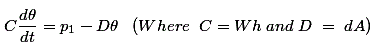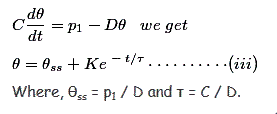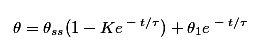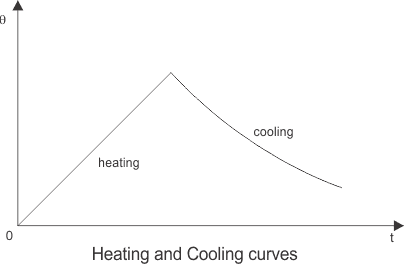# Thermal Model of a Motor

We know that when an electrical motor and drive operates, there is a generation of heat inside the motor. The amount of heat generated inside the motor should be known as accurately as possible. That’s why thermal modeling of motor is necessary. The material of the motors and the shapes and size of the motors are not unique but the generation of heat does not alter very much depending on these characteristics. So, a simple thermal model of any motor can be obtained assuming it to be a homogeneous body. The main aim of this modeling is to choose the appropriate rating of a motor so that the electric motor does not exceed its safe limit during operation.

At time ‘t’, let the motor has following parameters
p1 = Heat developed, Joules/sec or watts
p2 = Heat dissipated to the cooling medium, watts –
W = Weight of the active parts of the machine.
h = Specific heat, Joules per Kg per oC.
A = Cooling Surface, m2
d = Co-efficient of heat transfer, Joules/Sec/m2/oC
θ = Mean temperature rise oC

Now, if time dt, let the temperature rise of the machine be dθ,
Therefore, heat absorbed in the machine = (Heat generated inside the machine – Heat dissipated to the surrounding cooling medicine)
Where, dθ = p1dt – p2dt…………….(i)
Since, p2 = θdA…………….(ii)
Substituting (ii) in (i), we getHere, C is called the thermal capacity of the machine in watts/oC and D is the heat dissipation constant in watts/oC.

When we acquire the first order differential equation of the equation –We obtain the value of K by putting t = 0 in equation (iii) and get the solution asSo, from the above equation we can find out the rise in temperature inside a working machine, which is very near to being accurate and if we plot a graph for the variation of temperature risk with time during heating and cooling and thus the thermal modeling of a motor gets completed.Want To Learn Faster? 🎓
Get electrical articles delivered to your inbox every week.
No credit card required—it’s 100% free.